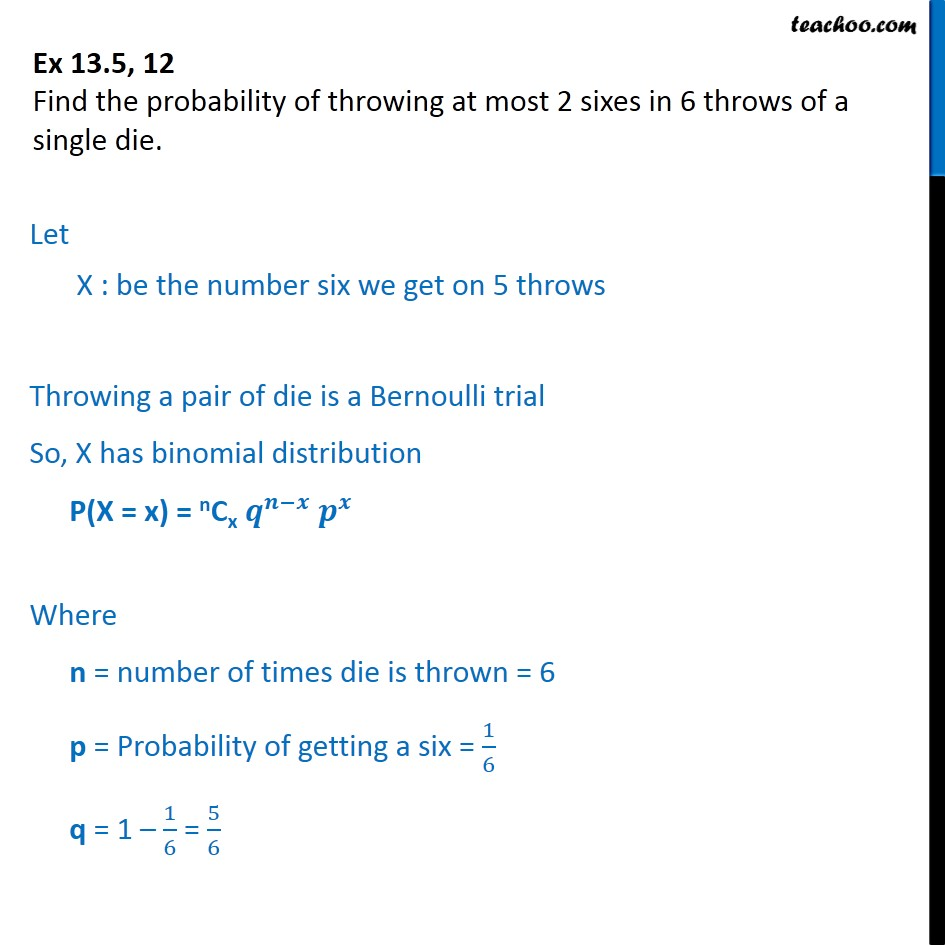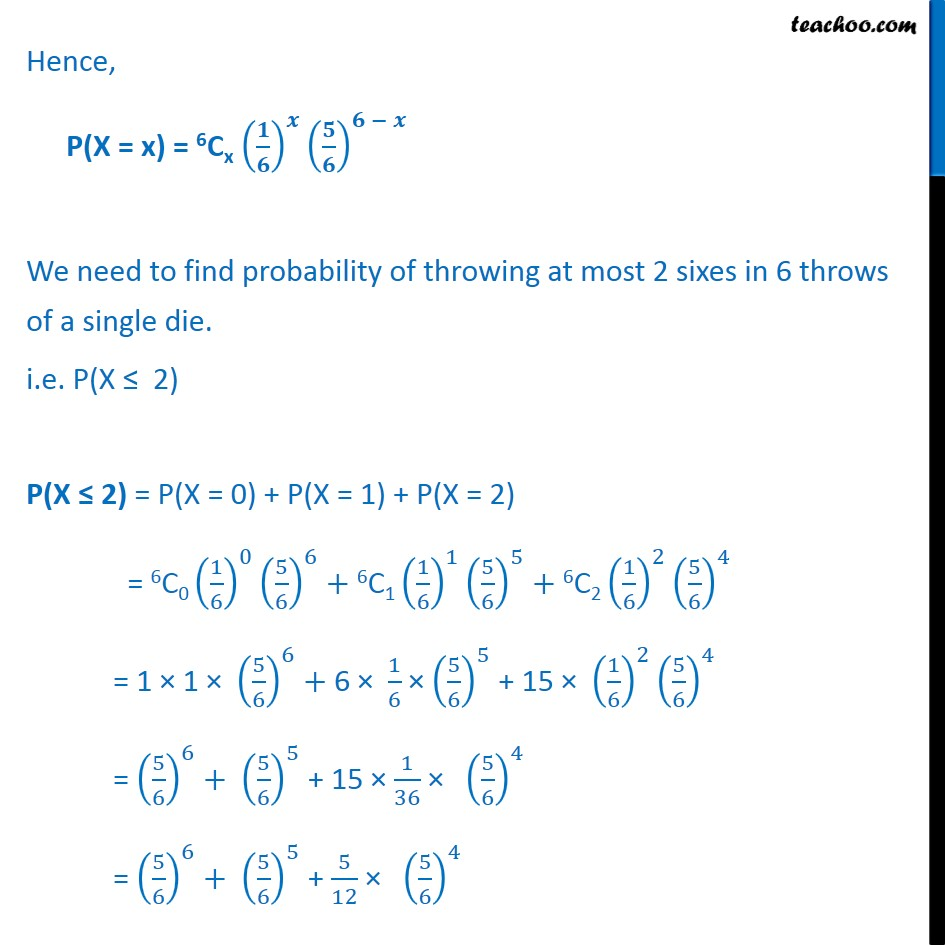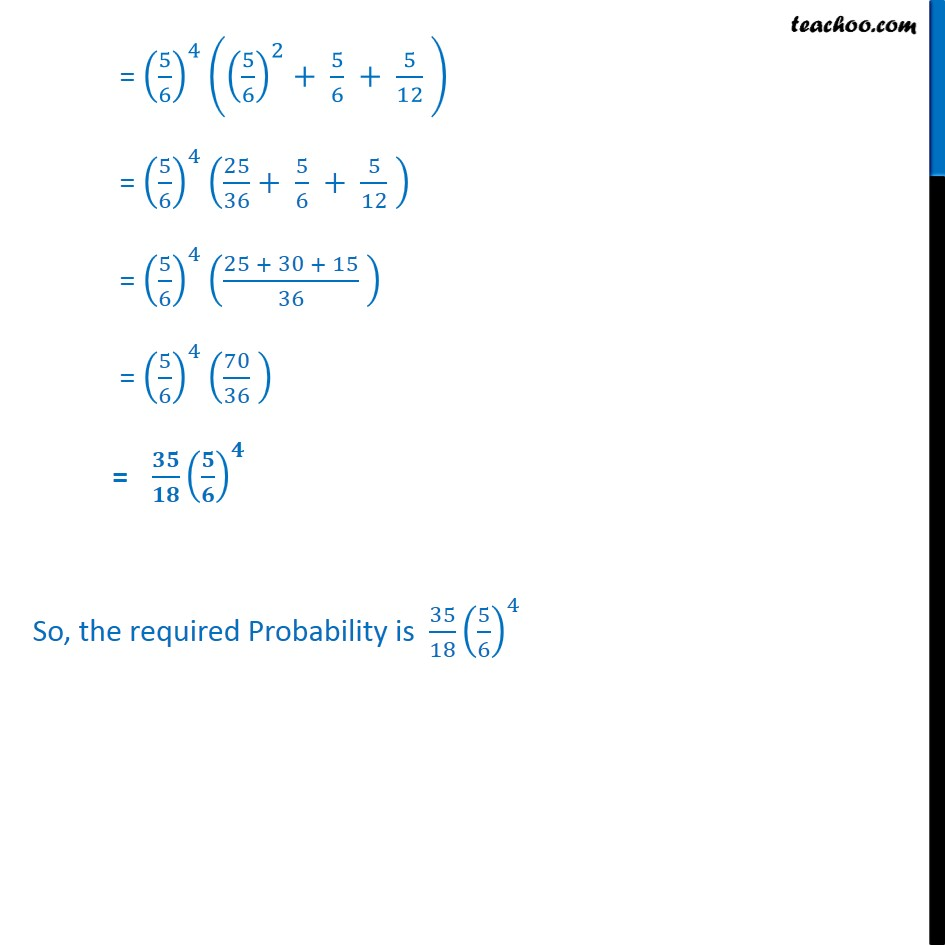Bernoulli Trial

Chapter 13 Class 12 Probability
Serial order wiseLearn in your speed, with individual attention - Teachoo Maths 1-on-1 Class

### Transcript

Question 12 Find the probability of throwing at most 2 sixes in 6 throws of a single die. Let X : be the number six we get on 5 throws Throwing a pair of die is a Bernoulli trial So, X has binomial distribution P(X = x) = nCx Where n = number of times die is thrown = 6 p = Probability of getting a six = 1 6 q = 1 1 6 = 5 6 Hence, P(X = x) = 6Cx We need to find probability of throwing at most 2 sixes in 6 throws of a single die. i.e. P(X 2) P(X 2) = P(X = 0) + P(X = 1) + P(X = 2) = 6C0 1 6 0 5 6 6 +6C1 1 6 1 5 6 5 +6C2 1 6 2 5 6 4 = 1 1 5 6 6 + 6 1 6 5 6 5 + 15 1 6 2 5 6 4 = 5 6 6 + 5 6 5 + 15 1 36 5 6 4 = 5 6 6 + 5 6 5 + 5 12 5 6 4 = 5 6 4 5 6 2 + 5 6 + 5 12 = 5 6 4 25 36 + 5 6 + 5 12 = 5 6 4 25 + 30 + 15 36 = 5 6 4 70 36 = So, the required Probability is 35 18 5 6 4